# Properties of Fractional and Negative Exponents

Author: Sophia Tutorial
##### Description:

Simplify an expression with fractional exponents.

(more)

Sophia’s self-paced online courses are a great way to save time and money as you earn credits eligible for transfer to many different colleges and universities.*

No credit card required

37 Sophia partners guarantee credit transfer.

299 Institutions have accepted or given pre-approval for credit transfer.

* The American Council on Education's College Credit Recommendation Service (ACE Credit®) has evaluated and recommended college credit for 32 of Sophia’s online courses. Many different colleges and universities consider ACE CREDIT recommendations in determining the applicability to their course and degree programs.

Tutorial
what's covered
1. Properties of Fractional and Negative Exponents

# 1. Properties of Fractional and Negative Exponents

When we simplify radicals with exponents, we divide the exponent by the index. Another way to write division is with a fraction bar. This idea is how we will define rational exponents.

formula
Property of Fractional Exponents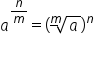The denominator of a rational exponent becomes the index on our radical. Likewise, the index on the radical becomes the denominator of the exponent. We can use this property to change any radical expression into an exponential expression.Index is denominatorNegative exponents from reciprocals

We can also change any rational exponent into a radical expression by using the denominator as the index.Index is denominatorNegative exponent means reciprocals

did you know
Nicole Oresme, a Mathematician born in Normandy was the first to use rational exponents. He used the notation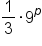to represent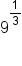. However, his notation went largely unnoticed.

The ability to change between exponential expressions and radical expressions allows us to evaluate problems we had no means of evaluating before by changing to a radical.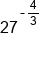Change to radical, denominator is index, negative means reciprocal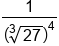Evaluate radical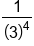Evaluate exponent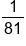Our Solution

The largest advantage of being able to change a radical expression into an exponential expression is we are now allowed to use all our exponent properties to simplify. The following table reviews all of our exponent properties.

big idea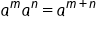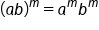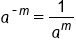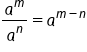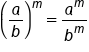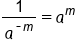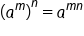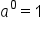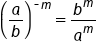When adding and subtracting with fractions, we need to be sure to have a common denominator. When multiplying, we only need to multiply the numerators together and denominators together. The following examples show several different problems, using different properties to simplify rational exponents.

EXAMPLENeed common denominator on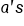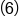and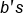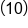Add exponents onand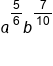Our Solution

EXAMPLEMultiply each exponent by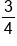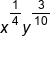Our Solution

EXAMPLEIn numerator, need common denominator to add exponents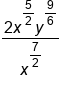Subtract exponents on x, reduce exponents on y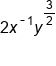Negative exponent moves down to denominator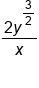Our Solution

EXAMPLENeed common denominator on x's in parentheses; use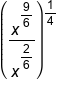Subtract exponents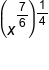Multiply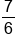by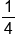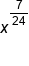Our Solution

summary
It is important to remember that as we simplify with fractional and negative exponents, we are using the same properties we used when simplifying integer exponents. The only difference is we need to follow our rules for fractions as well. It may be worth reviewing your notes on exponent properties to be sure you’re comfortable with using the properties.

Formulas to Know
Power of a Power Property of ExponentsPower of a Product Property of ExponentsPower of a Quotient Property of ExponentsProduct Property of ExponentsProperty of Fractional ExponentsQuotient Property of ExponentsRating